# Dynamic Named Ranges

You can create dynamic named ranges that automatically expand or contract depending on the number of items.
Most dynamic named ranges use the OFFSET function.
Other functions can then be used to obtain the necessary offset values.
Dynamic named ranges are not listed in the Name Box but you can type the name directly and press Enter to quickly select the cells.
There are lots of uses for dynamic named ranges and below are some of the most common:
1) Identify all the data in a particular column.
2) Identify only the numerical data in a particular column.
3) Identify a whole table (uniform).
4) Identify a whole table (not uniform)
5) Identify a whole table (with blanks)

### Example 1 - Identify all the data in a particular column

Using a named range to define the source data for a chart allows you to quickly add more data.
This example shows you how to create a named range that will contain a continuous block of non blank cells in a particular column.
Lets suppose that you wanted to create a chart for the following data.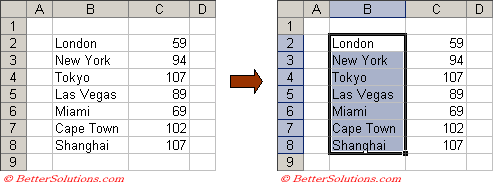In this example we want to be able to identify all the cells which contain data (i.e. that are not blank).
The COUNTA function returns the number of non blank cells in a cell range.
This function will also count any cells that contain an empty string "".
Select (Insert > Name > Define) and type the name "ChartLabels" in the Names in Workbook textbox.
Enter the following into the Refers To box:
=OFFSET(Sheet1!\$B\$2,0,0,COUNTA(Sheet1!\$B\$1:\$B\$100),1)
This formula uses the COUNTA() function to return the necessary height argument.
You do need to include the dollar signs \$ otherwise the correct formula will not be used.
You do not need to reference the whole of column B just the top 100 cells.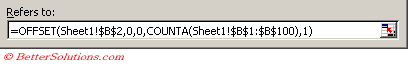Press OK to create the named range.
You can check that the named range got created by typing "ChartLabels" in the Name Box and pressing Enter.
To test that this named range is in fact dynamic type another city in cell "B9" and check the named range again.

### Example 2 - Identify only the numerical data in a particular column

The COUNT function returns the number of numeric values in a cell range.
If you wanted to create a dynamic named range that included a continuous block of only numeric cells (excluding any text after the numbers) you could use the COUNT function instead of the COUNTA function.
In this case the dates in column "B" are infact numbers so it would be appropriate to use the COUNT function.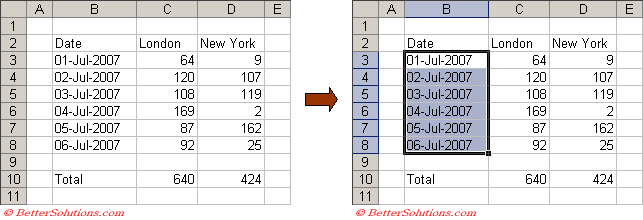Select (Insert > Name > Define) and type the name "Dates" in the Names in Workbook textbox.
Enter the following into the Refers To box:
=OFFSET(Sheet1!\$B\$3,0,0,COUNT(Sheet1!\$B\$1:\$B\$100),1)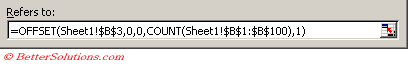You can check that the named range got created by typing "Dates" in the Name Box and pressing Enter.
To test this named range insert a row below row 8 and enter the date 07/07/2007.

### Example 3 - Identify a whole table

The previous two examples have defined the number of columns passed to the OFFSET function to be 1.
In this example we want to include the whole table so instead of passing in 1, we need to include the number of columns in the table.
If you know the exact number of columns in the table you can enter this value as the last argument.
If not you can use the COUNTA function in a similar way to return the number of columns in the table.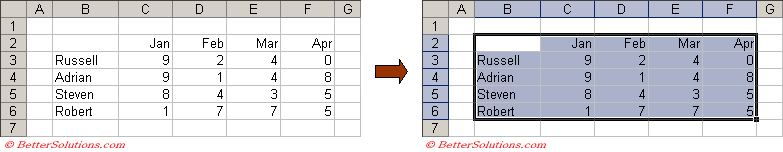Select (Insert > Name > Define) and type the name "WholeTable" in the Names in Workbook textbox.
Enter the following into the Refers To box:
=OFFSET(Sheet1!\$B\$2,0,0,COUNTA(Sheet1!\$C\$1:\$C\$100),COUNTA(Sheet1!\$A\$3:\$Z\$3))
This formula uses column "C" to obtain the necessary height and uses row 3 to obtain the necessary width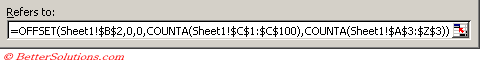You can check that the named range got created by typing "WholeTable" in the Name Box and pressing Enter.
To test this named range extend the table down to include another person and across to include May.

### Dynamic Named Ranges Alternatives / Examples

Offset(COUNT() )
Offset(COUNTA, ACOUNTA 0 - table
Offset(MATCH, ) - when your range includes blank cells MATCH(1E+300, \$A\$1:\$A\$1500,1)
The "1" at the end of the line above tells it to find the largest value less than or equal to position 1E+300

How many rows in a named range ?
If your named range refers to a single column

`Rows("NameRange_Column1") `

### Important

When editing the formulas in a named range do not use the arrow keys to manoeuvre around. Only use the mouse.
Avoid using the COUNTA function if you have formulas that might return an empty string ("").
If you do not use the dollar signs to represent absolute cell reference the column letters and row numbers are often changed randomly to other cell references.

### Example 4 - Identify a whole table (not uniform)

It is fairly common to have tables that are not uniform.
A non uniform table might contain different lengths for some of the columns.
If this is the case you need to identify which column contains the most items and use that to define the height of the table.
The easiest way to obtain the number of items in column "B" is to use the following formula:
=COUNTA(B3:B100)
Add an extra row above the table and insert these formulas across the top of each column, changing the column letter accordingly.Select (Insert > Name > Define) and type the name "UnEven" in the Names in Workbook textbox.
Enter the following into the Refers To box:
=OFFSET(Sheet1!\$B\$3,0,0,MAX(Sheet1!\$2:\$2),COUNTA(Sheet1!\$A\$3:\$Z\$3))
This formula uses the maximum value in the 2nd row as the height argument.

### Example 5 - Identify a whole table (with blanks)

In all the previous examples we have assumed that all the data is contained in a continuous block.
It is far more realistic to have a table that contains a combination of text and numbers as well as some blank cells.
When a table contains blank cells we cannot use either the COUNT or COUNTA functions.
The MATCH function returns a relative position of an item in a cell range in a specified order.
This function however can include blank cells so therefore can be used instead to obtain the last populated cell in each column.

You can also use INDIRECT